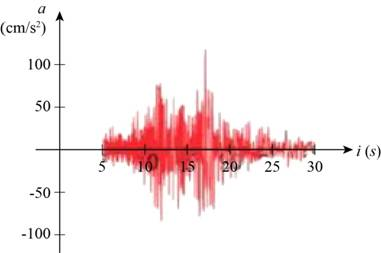# The time at which the earthquake first make the noticeable movements of the earth.### Precalculus: Mathematics for Calcu...

6th Edition
Stewart + 5 others
Publisher: Cengage Learning
ISBN: 9780840068071### Precalculus: Mathematics for Calcu...

6th Edition
Stewart + 5 others
Publisher: Cengage Learning
ISBN: 9780840068071

#### Solutions

Chapter 2.3, Problem 44E

(a)

To determine

## The time at which the earthquake first make the noticeable movements of the earth.

Expert Solution

The first noticeable movement took place after 5 seconds.

### Explanation of Solution

A graph is given which shows the vertical acceleration of the ground from an earthquake as measured by a seismograph.Figure (1)

The acceleration is given on vertical axis and the time in seconds on horizontal axis.

From Figure (1), it is noticed that the acceleration of ground is there right after 5 seconds.

Therefore, the first noticeable movement took place after 5 seconds.

(b)

To determine

### At what time the earthquake ended.

Expert Solution

The earthquake ended after 30 seconds.

### Explanation of Solution

A graph is given which shows the vertical acceleration of the ground from an earthquake as measured by a seismograph.Figure (1)

From Figure (1), it is noticed that there is no acceleration of the ground after 30 seconds. It clearly means that the earthquake stopped after 30 seconds.

Therefore, the earthquake ended in 30 seconds.

(c)

To determine

### At what time the earthquake had maximum intensity.

Expert Solution

The maximum intensity of the earthquake was reached after 17 seconds.

### Explanation of Solution

A graph is given which shows the vertical acceleration of the ground from an earthquake as measured by a seismograph.Figure (1)

Locate the local maximum point to find the time at which earthquake had the maximum intensity.

The value f(a) is local maximum value of function f if f(a)f(x) when a is near to x.

From the Figure (1), it is noticed that the value of acceleration is maximum between t=15 seconds and t=20 seconds.

The acceleration is more than 100cm/s2 at time near 17 seconds.

This is the point of local maximum.

Therefore, the maximum intensity of the earthquake was reached after 17 seconds.

### Have a homework question?

Subscribe to bartleby learn! Ask subject matter experts 30 homework questions each month. Plus, you’ll have access to millions of step-by-step textbook answers!